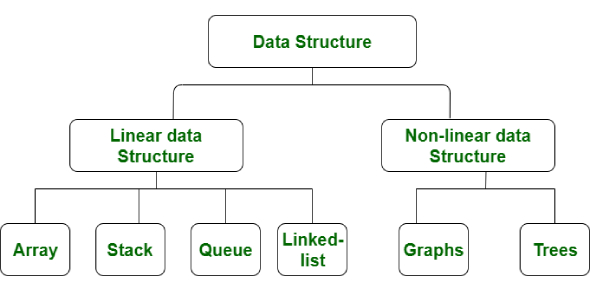# Data Structure Quiz Questions!

10 Questions | Attempts: 415
ShareSettingsWould you like a challenge? This quiz on data structure will blow your mind. With this quiz, you should know how to reverse the order of elements in and between positions, what the sequences do, what a single array is used for, and which permutations of printed values are possible. This quiz will help you understand data structure and enhance your knowledge. Give it a shot.

• 1.
Let the following circular queue can accommodate maximum six elements with the             following data             front = 2 rear = 4             queue = __ , L, M, N, ___, ___ What will happen after insert O operation takes place?
• A.

Front = 2 rear = 5 queue = __, L, M, N, O, ___

• B.

Front = 3 rear = 5 queue = __, L, M, N, O, ___

• C.

Front = 3 rear = 4 queue = __, L, M, N, O, ___

• D.

Front = 2 rear = 4         queue = L, M, N, O, ___

• 2.
Suppose you are given an array s[1...n] and a procedure reverse (s,i,j) which reverses the order of elements in a between positions i and j (both inclusive). What does the following sequence do, where 1 < k <= n: reverse (s, 1, k);reverse (s, k + 1, n);reverse (s, 1, n);
• A.

Rotates s left by k positions

• B.

Leaves s unchanged

• C.

Reverses all elements of s

• D.

None of the above

• 3.
A single array A[1..MAXSIZE] is used to implement two stacks. The two stacks grow from opposite ends of the array. Variables top1 and top2 (topl< top 2) point to the location of the topmost element in each of the stacks. If the space is to be used efficiently, the condition for “stack full” is
• A.

(top1 = MAXSIZE/2) and (top2 = MAXSIZE/2+1)

• B.

Top1 + top2 = MAXSIZE

• C.

(top1= MAXSIZE/2) or (top2 = MAXSIZE)

• D.

Top1= top2 -1

• 4.
What is a leaf node in a tree?
• A.

Node with 1 child

• B.

Node with n children

• C.

Node with zero child

• D.

None of these

• 5.
An item that is read as input can be either pushed to a stack and later popped and printed, or printed directly. Which of the following will be the output if the input is the sequence of items 1, 2, 3, 4, 5?
• A.

3, 4, 5, 1, 2

• B.

3, 4, 5, 2, 1

• C.

1, 5, 2, 3, 4

• D.

5, 4, 3, 1, 2

• 6.
Stack A has the entries a, b, c (with a on top), Stack B is empty. An entry popped out of stack A can be printed immediately or pushed to stack B. An entry popped out of stack B can only be printed. In the arrangement, which of the following permutations of printed values (a, b, c) is not possible?
• A.

B a c

• B.

B c a

• C.

C a b

• D.

A b c

• 7.
What does the following function do for a given Linked List? void fun1(struct Node* start) { if( start == NULL) return;   fun1(start->next); printf("%d ", start->data); }
• A.

Print the linked list from beginning

• B.

Print the linked list after first node

• C.

Print the first node only

• D.

None of these

• 8.
What does the following function do for a given Linked List? void fun2(struct Node* head) { if(start== NULL) return; printf("%d ", start->data);    if(start->next != NULL ) fun2(start->next->next); printf("%d ", start->data);  }
• A.

Print the linked list from beginning

• B.

Print the alternate node of the linked list

• C.

Print the first node only

• D.

None of the these

• 9.
A program P reads in 500 integers in the range [0..100] representing the scores of 500 students. It then prints the frequency of each score above 50. What would be the best way for P to store the frequencies?
• A.

An array of 50 numbers

• B.

An array of 100 numbers

• C.

An array of 500 numbers

• D.

A dynamically allocated array of 550 numbers

• 10.
Which one of the following is an application of Queue Data Structure?
• A.

When a resource is shared among multiple consumers

• B.

When data is transferred asynchronously (data not necessarily received at same rate as sent) between two processes

• C.Back to top